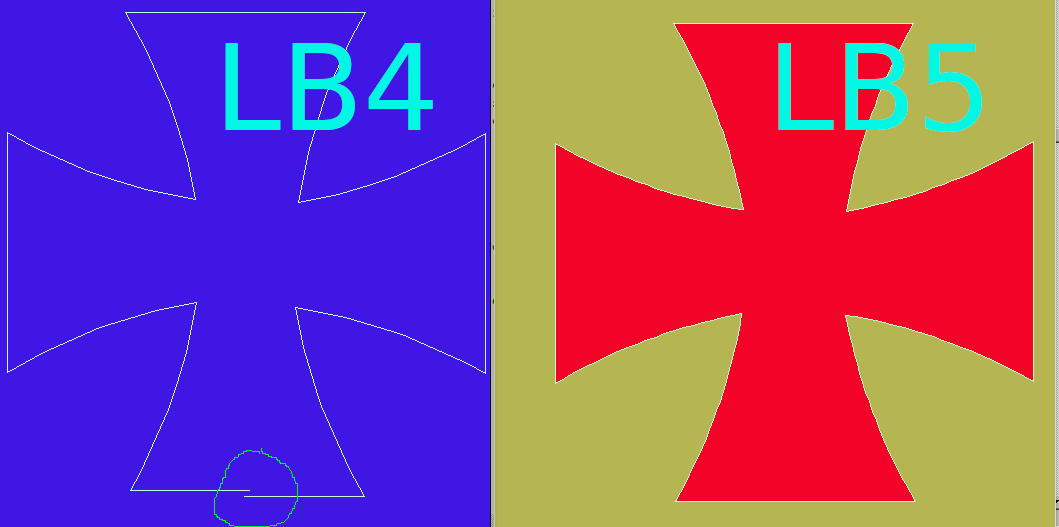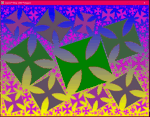# Maltese Cross## Code

In LB4 turtle graphics were useless for anything other than very simple- the turtle position and angle accumulated errors by being rounded off internally. LB5 seems much better, 'tho I've not deep-tested.

Here's a Maltese Cross. In LB4 the figure does not close. In 5 it does. So I can fill it with a colour- except dll calls now are not available ( ?yet?)-```
'   Maltese Cross curve

nomainwin

global pi, TX, TY, Ttheta
'TX  =250: TY =250: Ttheta =0 '   screen centre, pointing South/down.   '   <<<<<<<<<<<<<<<<<<<<<<<<<
pi  =4 *atn( 1)

WindowWidth  =502
WindowHeight =582

graphicbox #w.g, -100, -100, 800, 900

open "Maltese Cross" for graphics_nsb as #w

hw      =hwnd( #w.g)
calldll #user32, "GetDC", hw as ulong, hdc as ulong

#w "trapclose quit"

#w.g "down ; fill 180 180 80 ; size 1"

for p =1 to 200

F           =0.04 +0.2 *rnd( 1) '   sets scale

r           =int( 256 *rnd( 1))
g           =int( 256 *rnd( 1))
bl          =int( 256 *rnd( 1))

fillCol\$    =str\$( r) +" " +str\$( g) +" " +str\$( bl)

#w.g "color ";     fillCol\$
#w.g "backcolor "; fillCol\$

targetcolor   =bl *2^16    +g *2^8    +r      '   this is colour to fill WITH
'   this is also the colour of the outline to fill out TO.
TX =int( 0 +600 *rnd( 1)): TY =int( 100 +600 *rnd( 1)): Ttheta =-40 +80 *rnd( 1)

Cx  =TX    '   centre to fill from
Cy  =TY -5

for j =1 to 4
call forward 120 *F
call turn   -120
for i =1 to 20
call forward 7 *F
call turn    1
next i
call turn  110
for i =1 to 20
call forward 7 *F
call turn    1
next i
call turn   -120
call forward 120 *F
next j

call delay 1000

calldll #gdi32, "ExtFloodFill",_
hdc                as ulong,_
Cx                 as  long,_
Cy                 as  long,_
targetcolor        as  long,_                '   ie fill out 'til this colour is met..
_FLOODFILLBORDER   as  long,_
result             as  long

call delay 1000

next p

#w.g "getbmp scr 101 101 500 500"
bmpsave "scr", "MalteseCross" +str\$( time\$( "seconds")) +".bmp"

wait

sub delay sleeptime
'calldll #kernel32, "Sleep", sleeptime as long, ret as void
scan
end sub

sub quit h\$
calldll #user32, "ReleaseDC", hw as ulong, hdc as ulong, ret as void   'release the DC
close #w
end
end sub

'   <<<<<<<<<<<<<<<<<<<<<<<<<
end function

end function

sub draw lifted, x, y
if lifted =0 then #w "up" else #w "down"
#w.g "line "; TX; " "; TY; " "; x; " "; y
Ttheta  =atan2( x -TX, TY -y) *180 /pi  '   NB DEGREES.
TX      =x
TY      =y
end sub

sub turn angle  '   increment/update global turtle direction ( in DEGREES)
Ttheta =( Ttheta +angle)
if Theta <0 then Ttheta =Ttheta +360
Ttheta =Ttheta mod 360
end sub

sub forward s
#w.g "down ; line "; TX; " "; TY; " "; TX +dx; " "; TY +dy; " ; up"
TX =TX +dx
TY =TY +dy
end sub

function atan2( x, y)
Result\$ = "Undetermined"
If ( x = 0) and ( y > 0) Then atan2 = pi / 2:     Result\$ = "Determined"
If ( x = 0) and ( y < 0) Then atan2 = 3 * pi / 2: Result\$ = "Determined"
If ( x > 0) and ( y = 0) Then atan2 = 0:          Result\$ = "Determined"
If ( x < 0) and ( y = 0) Then atan2 = pi:         Result\$ = "Determined"
If Result\$ = "Determined" Then [End.of.function]
BaseAngle = Atn( abs( y) /abs( x))
If (x > 0) and (y > 0) Then atan2 =        BaseAngle
If (x < 0) and (y > 0) Then atan2 = pi    -BaseAngle
If (x < 0) and (y < 0) Then atan2 = pi    +BaseAngle
If (x > 0) and (y < 0) Then atan2 = 2*pi  -BaseAngle
[End.of.function]
end function
'   <<<<<<<<<<<<<<<<<<<<<<<<<
```